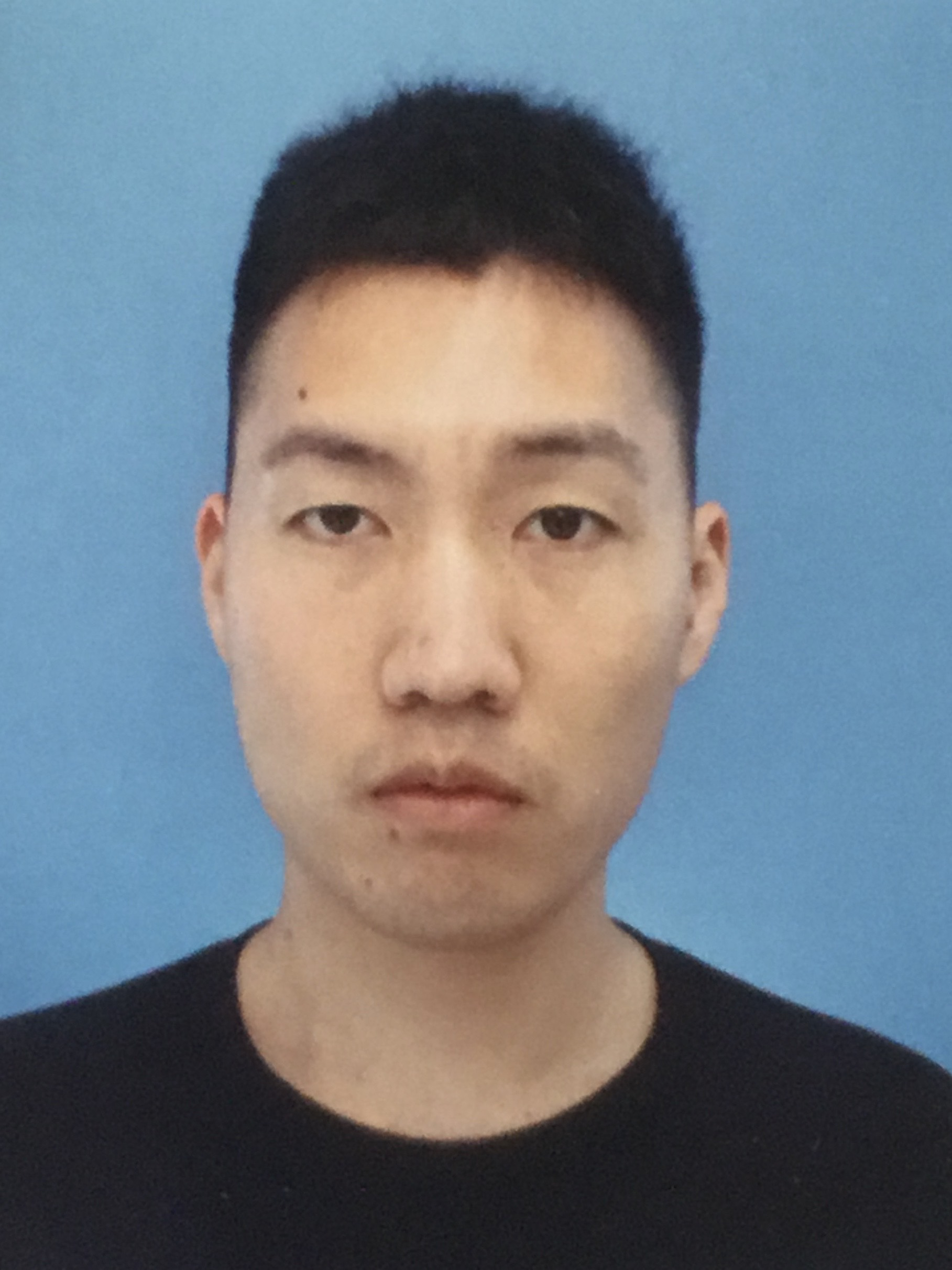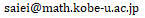Saiei-Jaeyeong MATSUBARA-HEO Saiei-Jaeyeong MATSUBARA-HEO Department of Mathematics, Graduate School of Science, Kobe University Division : Applied Mathematics Assistant Professor Building B, Room 309Research Field : Systems of linear partial differential (difference) equations in the complex domain, hypergeometric functions in several variables Research Summary : I study the theory of hypergeometric functions in several variables in the complex domain from the viewpoint of the theory of partial differential (difference) equations. Recently, I focus on Gelfand-Kapranov-Zelevinsky system and study its monodromy representation, connection problem, and asymptotic analysis. I also introduce and study a combinatorial structure on the intersection theory of a rapid decay (co)homology group with local system coefficients associated to an integral representation of GKZ system. Primary Publications : S.-J. Matsubara-Heo, N. Takayama, An algorithm of computing cohomology intersection number of hypergeometric integrals, arXiv:1904.01253. S.-J. Matsubara-Heo, Euler and Laplace integral representations of GKZ hypergeometric functions, arXiv:1904.0056. S.-J. Matsubara-Heo, On Mellin-Barnes integral representations for GKZ hypergeometric functions, Kyushu Journal of Mathematics, 74 (2020), 109--125. S.-J. Matsubara-Heo, Residue current approach to Ehrenpreis-Malgrange type theorem for linear differential equations with constant coefficients and commensurate time lags, Advances in Mathematics, 331 (2018), 170--208.